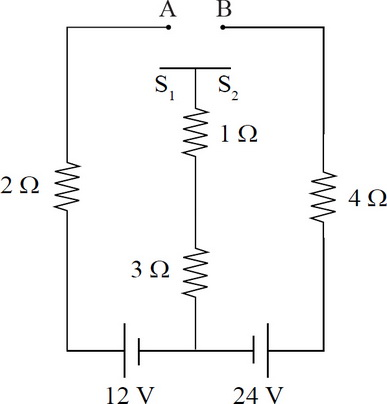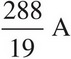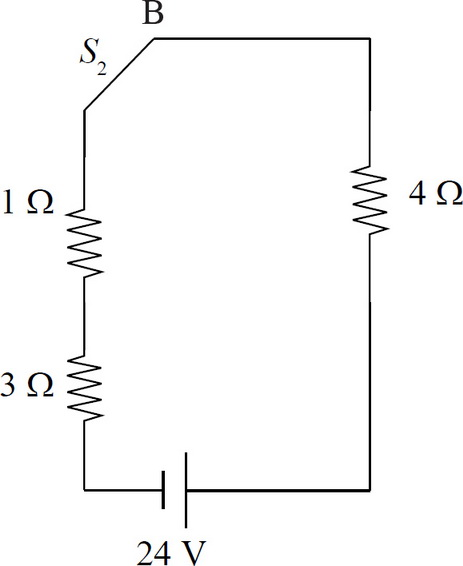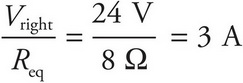# AP Physics 1 Question 298: Answer and Explanation

### Test Information

Question: 298

6. Question below refers to the below circuit diagram.If switch S2 is connected to point B but switch S1 is left unconnected, what is the current through the 1-Ω resistor?

• A. 0 A
• B. 2 A
• C. 3 A
• D.With S1 open but S2 closed, only the right-hand loop is connected. Again, the resistors are in series so the equivalent resistances is Req = 4 Ω + 1 Ω + 3 Ω = 8 Ω. The current through the circuit including the 1 Ω resistor is Iright =.